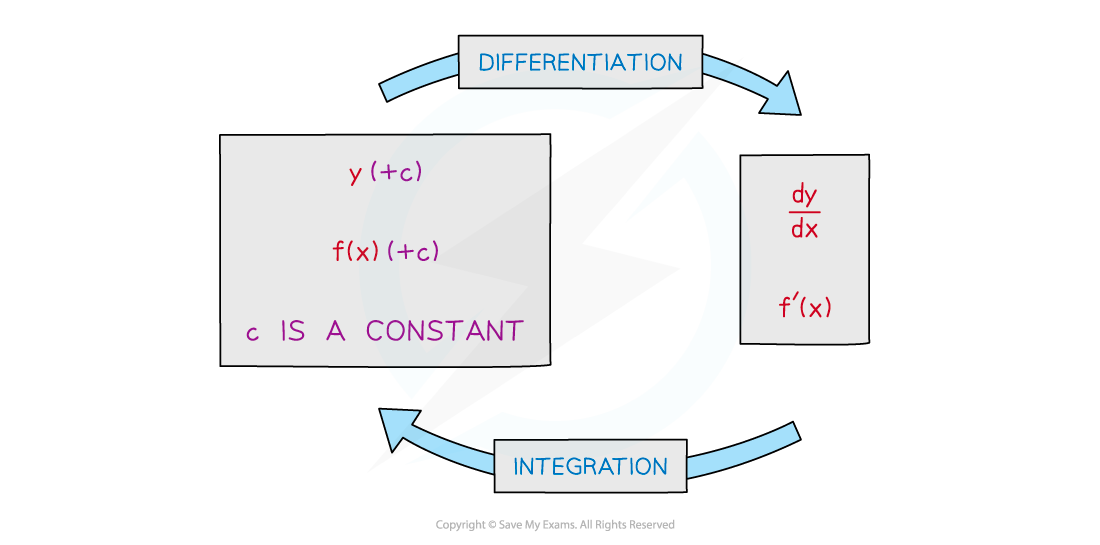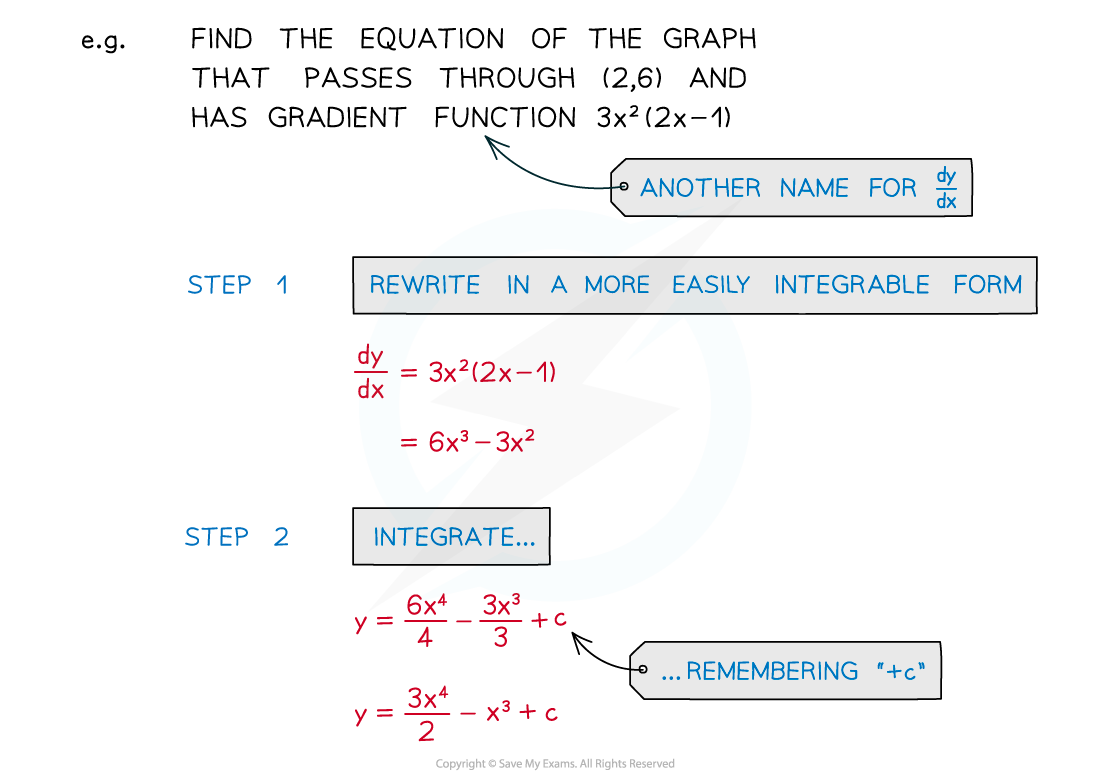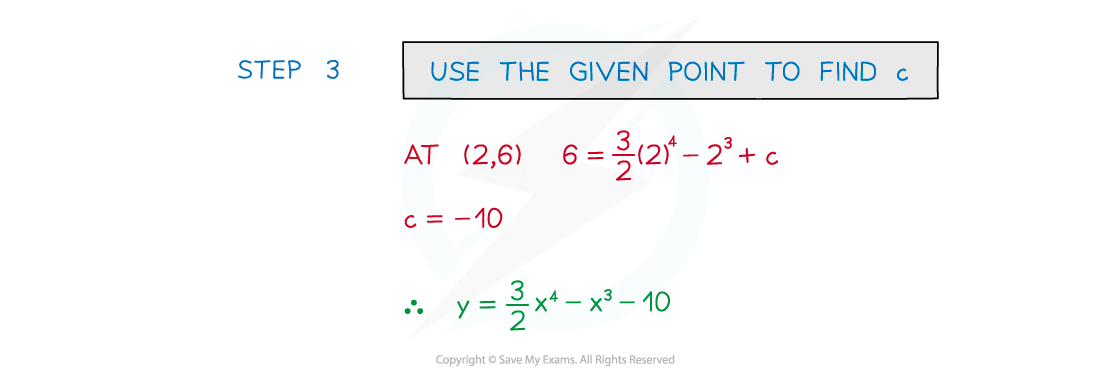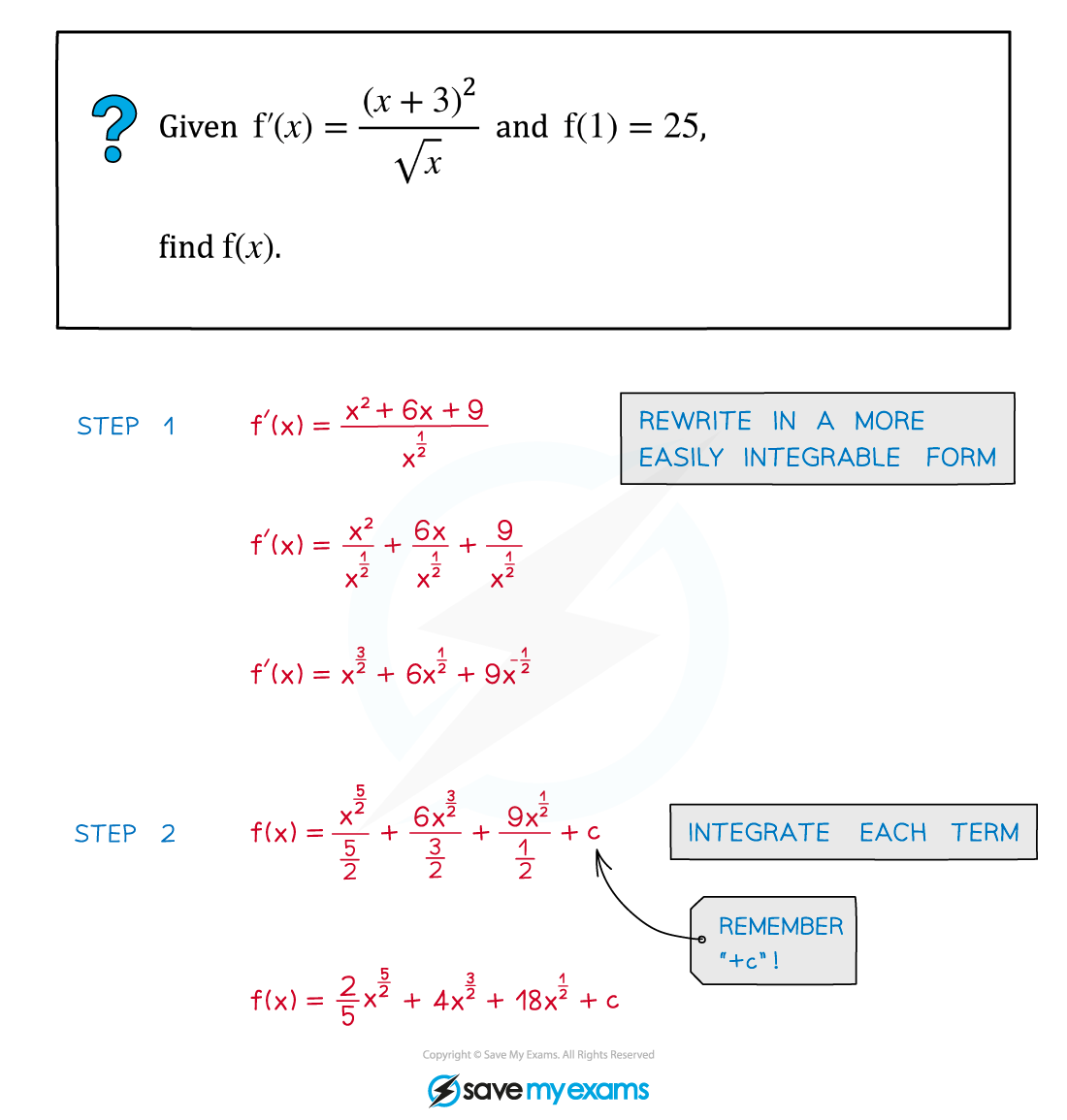# AQA A Level Maths: Pure复习笔记8.1.2 Integrating Powers of x

### Integrating Powers of x

#### What is integration?

•  Integration is the inverse ('opposite') of differentiation#### How do I integrate powers of x?•  For each term …
• … increase the power (of x) by 1
• … divide by the new power
• This does not apply when the original power is -1
• the new power would be 0 and division by 0 is undefined

#### How do I find c?

• STEP 1  Rewrite the function into a more easily integrable form
• Each term needs to be a power of x (or a constant)
• STEP 2  Integrate each term and remember “+c”
• Increase power by 1 and divide by new power
• STEP 3  Substitute the coordinates of a given point in to form an equation in c
• Solve the equation to find c#### Worked Example